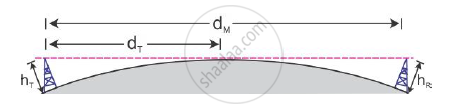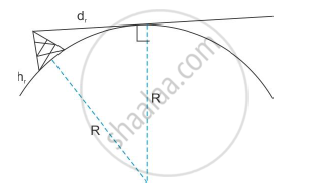Share

# Derive an Expression for the Maximum Line of Sight Distance Between Two Antennas for Space Wave Propagation. - CBSE (Science) Class 12 - Physics

ConceptPropagation of Electromagnetic Waves

#### Question

Derive an expression for the maximum line of sight distance between two antennas for space wave propagation.

#### Solution

Maximum line of sight distance:

The transmitting and receiving antennae are shown below.Consider only the transmitter antenna. From the geometry of the figure, we get(R+h_r)^2=R^2+d_r^2

:.R^2+2h_rR +h_r^2=R^2 +d_r^2

But, we know that h_r^2 "<< "2h_rR

∴ R^2+2h_rR=R^2+d_r^2

:.d_r^2=2h_rR

:.d_r=sqrt(2h_rR)

This is the distance between the transmitter and horizon.

Similarly, for the receiver, we have d_r=sqrt(2h_rR)

Hence, the maximum line-of-sight distance between the transmitter and the receiver is

d=d_r+d_R=sqrt(2Rh_r)+sqrt(2Rh_R)

Is there an error in this question or solution?

#### Video TutorialsVIEW ALL 

Solution Derive an Expression for the Maximum Line of Sight Distance Between Two Antennas for Space Wave Propagation. Concept: Propagation of Electromagnetic Waves.
S